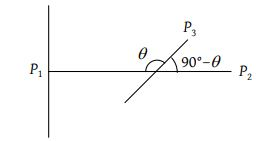# NEET Physics Wave Optics Questions Solved

Watch Physics > Wave Optics VideosTwo polaroids are placed in the path of unpolarized beam of intensity I0 such that no light is emitted from the second polaroid. If a third polaroid whose polarization axis makes an angle θ with the polarization axis of first polaroid, is placed between these polaroids then the intensity of light emerging from the last polaroid will be

(1) $\left(\frac{{I}_{0}}{8}\right){\mathrm{sin}}^{2}2\theta$

(2) $\left(\frac{{I}_{0}}{4}\right){\mathrm{sin}}^{2}2\theta$

(3) $\left(\frac{{I}_{0}}{2}\right){\mathrm{cos}}^{4}\theta$

(4) ${I}_{0}{\mathrm{cos}}^{4}\theta$

(1) No light is emitted from the second polaroid, so P1 and P2 are perpendicular to each otherLet the initial intensity of light is I0. So Intensity of light after transmission from first polaroid = $\frac{{I}_{0}}{2}$.

Intensity of light emitted from P3 ${I}_{1}=\frac{{I}_{0}}{2}{\mathrm{cos}}^{2}\theta$

Intensity of light transmitted from last polaroid i.e. from ${P}_{2}={I}_{1}{\mathrm{cos}}^{2}\left({90}^{o}-\theta \right)$ = $\frac{{I}_{0}}{2}{\mathrm{cos}}^{2}\theta .{\mathrm{sin}}^{2}\theta$

$=\frac{{I}_{0}}{8}{\left(2\mathrm{sin}\theta \mathrm{cos}\theta \right)}^{2}$ = $\frac{{I}_{0}}{8}{\mathrm{sin}}^{2}2\theta$.

Difficulty Level:

• 49%
• 25%
• 23%
• 6%
Crack NEET with Online Course - Free Trial (Offer Valid Till September 21, 2019)